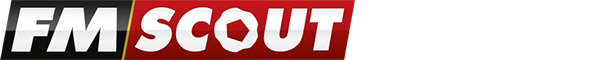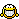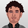On FM Scout you can chat about Football Manager in real time since 2011. Here are 10 reasons to join!

# New Coach Formula updated for Patch 9.3.0

On the way to 7 stars training

By Updated on Mar 03, 2009   58769 views   4 commentsAJetsOf on SI official forums posted a new Coach Formula updated for Patch 9.3.0.
9.3.0 Coach Formula: ddm = determination + discipline + motivating

strength, aerobics
fit * 9 + ddm * 2
>= 270 -> 7
>= 240 -> 6
>= 210 -> 5

goalkeeper
gk * 2 + ddm
>=90 -> 7
>=80 -> 6
>=70 -> 5

tactics
tac * 2 + ddm
>=90 -> 7
>=80 -> 6
>=70 -> 5

ball control
tec * 6 + men * 3 + ddm * 2
>=270 -> 7
>=240 -> 6
>=210 -> 5

defense
def * 8 + (tac + ddm) * 3
>=360 -> 7
>=320 -> 6
>=280 -> 5

attack
att * 6 + tac * 3 + ddm * 2
>=270 -> 7
>=240 -> 6
>=210 -> 5

shooting
tec * 6 + att * 3 + ddm * 2
>=270 -> 7
>=240 -> 6
>=210 -> 5

set pieces
(att + men + tec) * 3 + ddm * 2
>=270 -> 7
>=240 -> 6
>=210 -> 5
Example on how to get 7 stars training.

strength, aerobics
fit 20 + ddm>= 45 =7
fit 19 + ddm>= 50 =7
fit 18 + ddm>= 54 =7
fit 17 + ddm>= 59 =7

goalkeeper
gkp 20 + ddm>= 50 =7
gkp 19 + ddm>= 52 =7
gkp 18 + ddm>= 54 =7
gkp 17 + ddm>= 56 =7
gkp 16 + ddm>= 58 =7
gkp 15 + ddm>= 60 =7

tactics
tac 20 + ddm>= 50 =7
tac 19 + ddm>= 52 =7
tac 18 + ddm>= 54 =7
tac 17 + ddm>= 56 =7
tac 16 + ddm>= 58 =7
tac 15 + ddm>= 60 =7

defense
def 20 + (tac+ ddm)>=67 =7
def 19 + (tac+ ddm)>=70 =7
def 18 + (tac+ ddm)>=72 =7
def 17 + (tac+ ddm)>=75 =7
def 16 + (tac+ ddm)>=78 =7
def 15 + (tac+ ddm)>=80 =7

A more specific example
Some people have difficulty understanding the way I presented it, so here's an example:
Open Meulensteen in the data editor:

det=18 dis=18 mot=18
ddm=18+18+18=54
tac=15
att=18
def=12

Tactics Rating:
15 *2 + 54 = 84 -> 6 star

Attack Rating:
18 *6 + 15 * 3 + 54 *2 = 261 -> 6 star

Defense Rating:
12 * 8 + (15 + 54) * 3 = 303 -> 5 star

In a game where a coach (here I used Reulensteen as an example) has those stats, that's the training star rating they will get, as computed above. Depending on your luck, you might get a better or worse Reulensteen than the default setting.

Norwegian translation (thanks Hattijr)
Chinese translation (thanks ggamer)
German translation (thanks Octavianus[GER])

abojep on SI official forums created a spreadsheet that does all the maths for you. You just have to enter the coach details in the "Coaches" sheet and then look at the "Stars" sheet to see what rating it gives.

the_colonel on SI official forums created a spreadsheet where you put the stats for the coaches in and it tells you the star ratings, whether it's 7, 6, 5 or less than 5.

mmfaria on SI official forums developed an Excel sheet where you can put the coach's attributes and it returns how many stars he is at every training category.

##### Your content on FM Scout

We are always looking for quality content creators, capable of producing insightful articles. Being published here means more exposure and recognition for you.

Do YOU have what it takes?

## Discussion: New Coach Formula updated for Patch 9.3.0

4 comments have been posted so far.

•Fantastic!
•Cool, ta very much
•very useful thanks•ty
###### FMS Chat–Stam
hey, just wanted to let you know that we have a fb style chat for our members. login or sign up to start chatting.